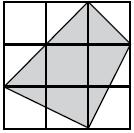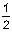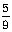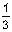# User Forum

Subject :NCO    Class : Class 4
Logical and Analytical Reasoning

What fraction of the given figure is shaded?(A)(B)(C)(D)please explain logic of answer B

Class : Class 3
4 and a half

## Ans 2:

Class : Class 5
1. First Row's Shaded Portion = 1 Square. 2. 2nd Row's 1st Square's 2nd Square's shaded portion = 1.5 Square. 3. 3rd Row's 1st Square's 2nd Square's shaded portion = 1 Square 4. 2nd Row's 3rd Square 3rd Row's 3rd Square's shaded portion = 1 Square So total 4.5 Square are there which is 1/2 of total 9 Square Hence, the Answer is : 1/2.

## Ans 3:

Class : Class 5
first row shaded area makes one square. last row first n second shaded area makes second square. third column last two shaded area makes third square. middle square is fourth shaded square. first column middle square is half shaded square. thus totoal shaded squares are 4.5 and divided by total number of squares, i.e. 9 equals 4.5/9＝1/2

## Ans 4:

Class : Class 2
first row shaded areamakes one square. last row first n second shaded area makes second square. third column last two shaded area makes third square. middle square is fourth shaded square. first column middle square is half shaded square. thus totoal shaded squares are 4.5 and divided by total number of squares, i.e. 9 equals 4.5/9＝1/2

## Ans 5:

Class : Class 4
1. First Row's Shaded Portion = 1 Square. 2. 2nd Row's 1st Square's+ 2nd Square's shaded portion = 1.5 Square. 3. 3rd Row's 1st Square's + 2nd Square's shaded portion = 1 Square 4. 2nd Row's 3rd Square + 3rd Row's 3rd Square's shaded portion = 1 Square So total 4.5 Square are there which is 1/2 of total 9 Square Hence, the Answer is : 1/2.